# Line-by-line module¶

This is the core of RADIS: it calculates the spectral densities for a homogeneous slab of gas, and returns a `Spectrum` object.

Calculations are performed within the `SpectrumFactory` class. `calc_spectrum()` is a high-level wrapper to `SpectrumFactory` for most simple cases.Cite all references used

Cite all references usedLine Survey

Line Survey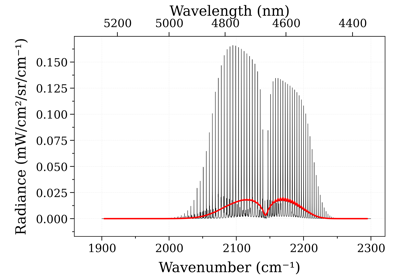Calculate non-LTE spectra of carbon-monoxide

Calculate non-LTE spectra of carbon-monoxide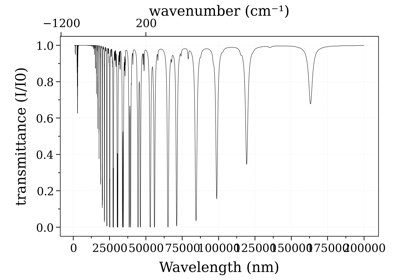Calculate a large spectrum by part

Calculate a large spectrum by partCompare CO spectrum from the GEISA and HITRAN database

Compare CO spectrum from the GEISA and HITRAN databaseCalculate a spectrum from HITEMP

Calculate a spectrum from HITEMP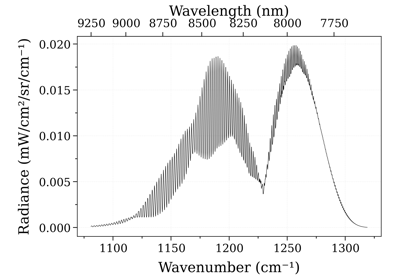Calculate a spectrum from ExoMol

Calculate a spectrum from ExoMol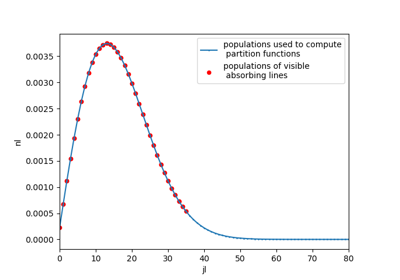See populations of computed levels

See populations of computed levelsCalculate a full range spectrum

Calculate a full range spectrumCompare CO xsections from the ExoMol and HITEMP database

Compare CO xsections from the ExoMol and HITEMP database

For any other question you can use the Q&A forum, the GitHub issues or the community chats on Gitter or Slack .## Line databases¶

List of supported line databases formats: `KNOWN_DBFORMAT`

### HITRAN¶

RADIS can automatically fetch HITRAN lines using the Astroquery module. This is done by specifying `databank=='hitran'` in `calc_spectrum()` or by using the `fetch_databank()` method in `SpectrumFactory`. Refer to `fetch_astroquery()` for more information.

You can also download the HITRAN databases files locally:

Note

RADIS has parsers to read line databases in Pandas dataframes. This can be useful if you want to edit the database. see `hit2df()`

There are also functions to get HITRAN molecule ids, and vice-versa: `get_molecule()`, `get_molecule_identifier()`

### HITEMP¶

RADIS can read files from the HITEMP database.

The `~/radis.json` is then used to properly handle the line databases on the User environment. See the Configuration file section, as well as the `radis.misc.config` module and the `getDatabankList()` function for more information.

📣 starting from radis==0.9.28 you can also download HITEMP directly. Example

```from radis import calc_spectrum
calc_spectrum(
wavenum_min=2500 / u.cm,
wavenum_max=4500 / u.cm,
molecule="OH",
Tgas=600,
databank="hitemp",  # test by fetching directly
verbose=False,
)
```
• Some HITEMP line databases are pre-configured in the RADIS-lab online environment. No install needed !

• If you just want to parse the HITEMP files, use `fetch_hitemp()`

```from radis.io.hitemp import fetch_hitemp
fetch_hitemp("NO")
```

### CDSD-4000¶

RADIS can read files from the CDSD-4000 database, however files have to be downloaded manually.

The `~/radis.json` is used to properly handle the line databases on the User environment. See the Configuration file section, as well as the `radis.misc.config` module and the `getDatabankList()` function for more information.

Note

See `cdsd2df()` for the conversion to a Pandas DataFrame.

## Calculating spectra¶

### Calculate one molecule spectrum¶

In the following example, we calculate a CO spectrum at equilibrium from the latest HITRAN database, and plot the transmittance:

```s = calc_spectrum(
wavenum_min=1900,
wavenum_max=2300,
Tgas=700,
path_length=0.1,
molecule='CO',
mole_fraction=0.5,
isotope=1,
wstep=0.01,
databank='hitran'   # or 'hitemp'
)
s.plot('transmittance_noslit')
```

### Calculate multiple molecules spectrum¶

RADIS can also calculate the spectra of multiple molecules. In the following example, we add the contribution of CO2 and plot the transmittance:

```s = calc_spectrum(
wavenum_min=1900,
wavenum_max=2300,
Tgas=700,
path_length=0.1,
mole_fraction={'CO2':0.5, 'CO':0.5},
wstep=0.01,
isotope=1,
)
s.plot('transmittance_noslit')
```

Note that you can indicate the considered molecules either as a list in the `molecule` parameter, or in `isotope` or `mole_fraction`. The following commands give the same result:

```# Give molecule:
s = calc_spectrum(
wavelength_min=4165,
wavelength_max=5000,
Tgas=1000,
path_length=0.1,
molecule=["CO2", "CO"],
mole_fraction=1,
isotope={"CO2": "1,2", "CO": "1,2,3"},
wstep=0.01,
verbose=verbose,
)

# Give isotope only
s = calc_spectrum(
wavelength_min=4165,
wavelength_max=5000,
Tgas=1000,
path_length=0.1,
isotope={"CO2": "1,2", "CO": "1,2,3"},
verbose=verbose,
)

# Give mole fractions only
s = calc_spectrum(
wavelength_min=4165,
wavelength_max=5000,
Tgas=1000,
path_length=0.1,
mole_fraction={"CO2": 0.2, "CO": 0.8},
isotope="1,2",
verbose=verbose,
)
```

Be careful to be consistent and not to give partial or contradictory inputs.

```# Contradictory input:
s = calc_spectrum(
wavelength_min=4165,
wavelength_max=5000,
Tgas=1000,
path_length=0.1,
mole_fraction=1,
isotope={"CO2": "1,2", "CO": "1,2,3"},
verbose=verbose,
)

# Partial input:
s = calc_spectrum(
wavelength_min=4165,
wavelength_max=5000,
Tgas=1000,
path_length=0.1,
molecule=["CO2", "CO"],  # contradictory
mole_fraction=1,
isotope={"CO2": "1,2"},  # unclear for CO
verbose=verbose,
)
```

### Equilibrium Conditions¶

By default RADIS calculates spectra at thermal equilibrium (one temperature).

The `calc_spectrum()` function requires a given mole fraction, which may be different from chemical equilibrium.

You can also compute the chemical equilibrium composition in other codes like [CANTERA], and feed the output to RADIS `calc_spectrum()`. The `get_eq_mole_fraction()` function provides an interace to [CANTERA] directly from RADIS

```from radis import calc_spectrum, get_eq_mole_fraction

# calculate gas composition of a 50% CO2, 50% H2O mixture at 1600 K:
gas = get_eq_mole_fraction('CO2:0.5, H2O:0.5', 1600, # K
1e5  # Pa
)
# calculate the contribution of H2O to the spectrum:
calc_spectrum(...,
mole_fraction=gas['H2O']
)
```

### Nonequilibrium Calculations¶

Non-LTE calculations (multiple temperatures) require to know the vibrational and rotational energies of each level in order to calculate the nonequilibrium populations.

You can either let RADIS calculate rovibrational energies with its built-in spectroscopic constants, or supply an energy level database. In the latter case, you need to edit the Configuration file .

### Calculating spectrum using GPU¶

RADIS also supports CUDA-native parallel computation, specifically for lineshape calculation and broadening. To use these GPU-accelerated methods to compute the spectra, use either `calc_spectrum()` function with parameter `mode` set to `gpu`, or `eq_spectrum_gpu()`. In order to use these methods, ensure that your system has an Nvidia GPU with compute capability of atleast 3.0 and CUDA Toolkit 8.0 or above. Refer to GPU Spectrum Calculation on RADIS to see how to setup your system to run GPU accelerated spectrum calculation methods, examples and performance tests.

Currently, GPU-powered spectra calculations are supported only at thermal equilibrium and therefore, the method to calculate the spectra has been named `eq_spectrum_gpu()`. In order to use this method to calculate the spectra, follow the same steps as in the case of a normal equilibrium spectra, and if using `calc_spectrum()` function set the parameter `mode` to `gpu`, or use `eq_spectrum_gpu()`

One could compute the spectra with the assistance of GPU using the following code as well

```s = calc_spectrum(
wavenum_min=1900,
wavenum_max=2300,
Tgas=700,
path_length=0.1,
mole_fraction=0.01,
isotope=1,
mode='gpu'
)
```

Refer to GPU Spectrum Calculation on RADIS for more details.

## Under the hood¶

### Flow Chart¶

RADIS can calculate populations of emitting/absorbing levels by scaling tabulated data (equilibrium) or from the rovibrational energies (nonequilibrium), get the emission and absorption coefficients from Line Databases, calculate the line broadening using various strategies to improve Performances, and produce a Spectrum object. These steps can be summarized in the flow chart below:The detail of the functions that perform each step of the RADIS calculation flow chart is given in Architecture.

### The Spectrum Factory¶

Most RADIS calculations can be done using the `calc_spectrum()` function. Advanced examples require to use the `SpectrumFactory` class, which is the core of RADIS line-by-line calculations. `calc_spectrum()` is a wrapper to `SpectrumFactory` for the simple cases.

The `SpectrumFactory` allows you to :

• calculate multiple spectra (batch processing) with a same line database

• edit the line database manually

• have access to intermediary calculation variables

• connect to a database of precomputed spectra on your computer

To use the `SpectrumFactory`, first load your own line database with `load_databank()`, and then calculate several spectra in batch using `eq_spectrum()` and `non_eq_spectrum()`, and `units`

```import astropy.units as u
from radis import SpectrumFactory
sf = SpectrumFactory(wavelength_min=4165 * u.nm,
wavelength_max=4200 * u.nm,
path_length=0.1 * u.m,
pressure=20 * u.mbar,
molecule='CO2',
wstep = 0.01,
isotope='1,2',
cutoff=1e-25,              # cm/molecule
)
sf.load_databank('HITRAN-CO2-TEST', load_columns='noneq')        # this database must be defined in ~/radis.json
s1 = sf.eq_spectrum(Tgas=300 * u.K)
s2 = sf.eq_spectrum(Tgas=2000 * u.K)
s3 = sf.non_eq_spectrum(Tvib=2000 * u.K, Trot=300 * u.K)
```

Note that for non-LTE calculations, specific columns must be loaded. This is done by using the `load_columns='noneq'` parameter. See `load_databank()` for more information.

### Configuration file¶

The `~/radis.json` configuration file is used to initialize your `radis.config`. It will :

• store the list and attributes of the Line databases available on your computer.

• change global user preferences, such as plotting styles and libraries, or warnings thresholds, or default algorithms.

The list of all available parameters is given in the default_radis.json file. Any key added to your `~/radis.json` will override the value of `default_radis.json`.

Note

You can also update config parameters at runtime by setting:

```import radis
```

Although it is recommended to simply edit your `~/radis.json` file.

Databases downloaded from ‘hitran’, ‘hitemp’ and ‘exomol’ with `calc_spectrum()` or `fetch_databank()` are automatically registered in the `~/radis.json` configuration file. The default download path is `~/.radisdb`. You can change this at runtime by setting the `radis.config["DEFAULT_DOWNLOAD_PATH"]` key, or (recommended) by adding a `DEFAULT_DOWNLOAD_PATH` key in your `~/radis.json` configuration file.

The configuration file will help to:

• handle local line databases that contains multiple files

• use custom tabulated partition functions for equilibrium calculations

• use custom, precomputed energy levels for nonequilibrium calculations

Note

it is also possible to work with local line databases without a configuration file, either by giving a file to the `databank=...` parameter of `calc_spectrum()` , or by giving to `load_databank()` the line database path, format, and partition function format directly.

However, this is not recommended and should only be used if for some reason you cannot create a configuration file.

A `~/radis.json` is user-dependant, and machine-dependant. It contains a list of database, each of which is specific to a given molecule. It typically looks like:

str: Typical expected format of a ~/radis.json entry:

```{
"database": {                                     # database key: all databanks information are stored in this key
"MY-HITEMP-CO2": {                            # your databank name: use this in calc_spectrum()
"path": [                                   # no "", multipath allowed
"D:\\Databases\\HITEMP-CO2\\hitemp_07",
"D:\\Databases\\HITEMP-CO2\\hitemp_08",
"D:\\Databases\\HITEMP-CO2\\hitemp_09"
],
"format": "hitran",                         # 'hitran' (HITRAN/HITEMP), 'cdsd-hitemp', 'cdsd-4000'
# databank text file format. More info in
"parfuncfmt": "hapi"                        # calculate partition functions
}
}
}
```

Following is an example where the path variable uses a wildcard `*` to find all the files that have `hitemp_*` in their names:

```{
"database": {                                     # database key: all databanks information are stored in this key
"MY-HITEMP-CO2": {                            # your databank name: use this in calc_spectrum()
"path": "D:\\Databases\\HITEMP-CO2\\hitemp_*",   # To load all hitemp files directly
"format": "hitran",                         # 'hitran' (HITRAN/HITEMP), 'cdsd-hitemp', 'cdsd-4000'
# databank text file format. More info in
"parfuncfmt": "hapi"                        # calculate partition functions
}
}
}
```

In the former example, for equilibrium calculations, RADIS uses [HAPI] to retrieve partition functions tabulated with TIPS-2017. It is also possible to use your own partition functions, for instance:

```{
"database": {                                       # database key: all databanks information are stored in this key
"MY-HITEMP-CO2": {                              # your databank name: use this in calc_spectrum()
"path": [                                     # no "", multipath allowed
"D:\\Databases\\HITEMP-CO2\\hitemp_07",
"D:\\Databases\\HITEMP-CO2\\hitemp_08",
"D:\\Databases\\HITEMP-CO2\\hitemp_09"
],
"format": "hitran",                           # 'hitran' (HITRAN/HITEMP), 'cdsd-hitemp', 'cdsd-4000'
# databank text file format. More info in
"parfuncfmt": "cdsd",                         # 'cdsd', 'hapi', etc.
# format to read tabulated partition function
# file. If `hapi`, then HAPI (HITRAN Python
# interface) is used to retrieve them (valid if
# your databank is HITRAN data). HAPI is embedded
# into RADIS. Check the version. If not specified then 'hapi'
# is used as default
"parfunc": "PATH/TO/cdsd_partition_functions.txt"
# path to tabulated partition function to use.
# If `parfuncfmt` is `hapi` then `parfunc`
# should be the link to the hapi.py file. If
# not given, then the hapi.py embedded in RADIS
# is used (check version)
}
}
}
```

By default, for nonequilibrium calculations, RADIS built-in spectroscopic constants are used to calculate the energy levels for CO2. It is also possible to use your own Energy level database. For instance:

```{
"database": {                                        # database key: all databanks information are stored in this key
"MY-HITEMP-CO2": {                               # your databank name: use this in calc_spectrum()
"path": [                                      # no "", multipath allowed
"D:\\Databases\\HITEMP-CO2\\hitemp_07",
"D:\\Databases\\HITEMP-CO2\\hitemp_08",
"D:\\Databases\\HITEMP-CO2\\hitemp_09"
],
"format": "hitran",                             # 'hitran' (HITRAN/HITEMP), 'cdsd-hitemp', 'cdsd-4000'
# databank text file format. More info in
# is used (check version)
"levels_iso1": "D:\\PATH_TO\\energies_of_626_isotope.levels",
"levels_iso2": "D:\\PATH_TO\\energies_of_636_isotope.levels",
"levelsfmt": "cdsd",                            # 'cdsd', etc.
# how to read the previous file. Default None.
"levelszpe": "2531.828"                         # zero-point-energy (cm-1): offset for all level
# energies. Default 0 (if not given)
}
}
}
```

The full description of a `json` entry is given in `DBFORMAT`:

• `path` corresponds to Line databases (here: downloaded from [HITEMP-2010]) and the `levels_iso` are user generated Energy databases (here: calculated from the [CDSD-4000] Hamiltonian on non-distributed code, which takes into account non diagonal coupling terms).

• `format` is the databank text file format. It can be one of `'hitran'` (for HITRAN / HITEMP 2010), `'cdsd-hitemp'` and `'cdsd-4000'` for the different CDSD versions (for CO2 only). See full list in `KNOWN_DBFORMAT`.

• `parfuncfmt`: `cdsd`, `hapi` is the format of the tabulated partition functions used. If `'hapi'`, then [HAPI] is used to retrieve them (valid if your databank is HITRAN data). See full list in `KNOWN_PARFUNCFORMAT`

• `parfunc` is the path to the tabulated partition function to use in in equilibrium calculations (`eq_spectrum()`). If `parfuncfmt` is `'hapi'` then `parfunc` should be the link to the hapi.py file. If not given, then the `hapi` embedded in RADIS is used (check version)

• `levels_iso#` are the path to the energy levels to use for each isotope, which are needed for nonequilibrium calculations (`non_eq_spectrum()`).

• `levelsfmt` is the energy levels database format. Typically, `'radis'`, and various implementation of [CDSD-4000] nonequilibrium partitioning of vibrational and rotational energy: `'cdsd-pc'`, `'cdsd-pcN'`, `'cdsd-hamil'`. See full list in `KNOWN_LVLFORMAT`

How to create the configuration file?

A default `~/radis.json` configuration file can be generated with `setup_test_line_databases()`, which creates two test databases from fragments of [HITRAN-2020] line databases:

```from radis.test.utils import setup_test_line_databases
setup_test_line_databases()
```

which will create a `~/radis.json` file with the following content

```{
"database": {
"HITRAN-CO2-TEST": {
"info": "HITRAN 2016 database, CO2, 1 main isotope (CO2-626), bandhead: 2380-2398 cm-1 (4165-4200 nm)",
"format": "hitran",
"parfuncfmt": "hapi",
},
"HITRAN-CO-TEST": {
"info": "HITRAN 2016 database, CO, 3 main isotopes (CO-26, 36, 28), 2000-2300 cm-1",
"format": "hitran",
"parfuncfmt": "hapi",
},
"HITEMP-CO2-TEST": {
"info": "HITEMP-2010, CO2, 3 main isotope (CO2-626, 636, 628), 2283.7-2285.1 cm-1",
"format": "cdsd-hitemp",
"parfuncfmt": "hapi",
}
}
}
```

If you configuration file exists already, the test databases will simply be appended.

### Calculation Flow Chart¶

Refer to Architecture for an overview of how equilibrium and nonequilibrium calculations are conducted.

### Use Custom Spectroscopic constants¶

Spectroscopic constants are a property of the RADIS `ElectronicState` class. All molecules are stored in the `Molecules` dictionary. You need to update this dictionary before running your calculation in order to use your own spectroscopic constants.

An example of how to use your own spectroscopic constants:

```from radis import calc_spectrum
from radis.db.molecules import Molecules, ElectronicState

Molecules['CO2']['X'] = ElectronicState('CO2', isotope=1, state='X', term_symbol='1Σu+',
spectroscopic_constants='my_constants.json',  # <<< YOUR FILE HERE
spectroscopic_constants_type='dunham',
Ediss=44600,
)
s = calc_spectrum(...)
```Calculate Rovibrational Energies

Calculate Rovibrational Energies

### Vibrational bands¶

To calculate vibrational bands of a given spectrum separately (vibrational-state-specific calculations), use the `eq_bands()` and `non_eq_bands()` methods. See the `test_plot_all_CO2_bandheads()` example in `radis/test/lbl/test_bands.py` for more information.

### Connect to a Spectrum Database¶

In RADIS, the same code can be used to retrieve precomputed spectra if they exist, or calculate them and store them if they don’t. See Precompute Spectra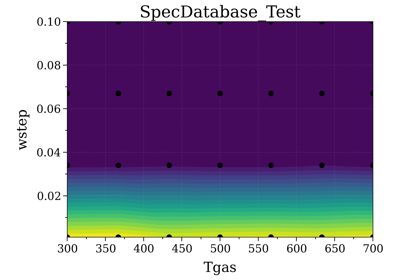Spectrum Database

Spectrum Database

## Performance¶

RADIS is very optimized, making use of C-compiled libraries (NumPy, Numba) for computationally intensive steps, and data analysis libraries (Pandas) to handle lines databases efficiently. Additionaly, different strategies and parameters are used to improve performances further:

### Line Database Reduction Strategies¶

By default:

• linestrength cutoff : lines with low linestrength are discarded after the new populations are calculated. Parameter: `cutoff` (see the default value in the arguments of `eq_spectrum()`)

Additional strategies (deactivated by default):

• weak lines (pseudo-continuum): lines which are close to a much stronger line are called weak lines. They are added to a pseudo-continuum and their lineshape is calculated with a simple rectangular approximation. See the default value in the arguments of `pseudo_continuum_threshold` (see arguments of `eq_spectrum()`)

### Lineshape optimizations¶

Lineshape convolution is usually the performance bottleneck in any line-by-line code.

Two approaches can be used:

• improve the convolution efficiency. This involves using an efficient convolution algorithm, using a reduced convolution kernel, analytical approximations, or multiple spectral grid.

• reduce the number of convolutions (for a given number of lines): this is done using the LDM strategy.

RADIS implements the two approaches as well as various strategies and parameters to calculate the lineshapes efficiently.

• broadening width : lineshapes are calculated on a reduced spectral range. Voigt computation calculation times scale linearly with that parameter. Gaussian x Lorentzian calculation times scale as a square with that parameter. parameters: broadening_max_width

• Voigt approximation : Voigt is calculated with an analytical approximation. Parameter : `broadening_max_width` and default values in the arguments of `eq_spectrum()`. See `voigt_lineshape()`.

• Fortran precompiled : previous Voigt analytical approximation is precompiled in Fortran to improve performance times. This is always the case and cannot be changed on the user side at the moment. See the source code of `voigt_lineshape()`.

• Multiple spectral grids : many LBL codes use different spectral grids to calculate the lineshape wings with a lower resolution. This strategy is not implemented in RADIS.

• LDM : lines are projected on a Lineshape database to reduce the number of calculated lineshapes from millions to a few dozens. With this optimization strategy, the lineshape convolution becomes almost instantaneous and all the other strategies are rendered useless. Projection of all lines on the lineshape database becomes the performance bottleneck. parameters: `ldm_res_L`, `ldm_res_G`. (this is the default strategy implemented in RADIS). Learn more in [Spectral-Synthesis-Algorithm]

More details on the parameters below:

### Computation parameters¶

If performance is an issue (for instance when calculating polyatomic spectra on large spectral ranges), you may want to tweak the computation parameters in `calc_spectrum()` and `SpectrumFactory`. In particular, the parameters that have the highest impact on the calculation performances are:

• The `broadening_max_width`, which defines the spectral range over which the broadening is calculated.

• The linestrength `cutoff`, which defines which low intensity lines should be discarded. See `plot_linestrength_hist()` to choose a correct cutoff.

Check the [RADIS-2018] article for a quantitative assessment of the influence of the different parameters.

Other strategies are possible, such as calculating the weak lines in a pseudo-continuum. This can result in orders of magnitude improvements in computation performances.:

• The `pseudo_continuum_threshold` defines which treshold should be used.

See the `test_abscoeff_continuum()` case in `radis/test/lbl/test_broadening.py` for an example, which can be run with (you will need the CDSD-HITEMP database installed)

```pytest radis/test/lbl/test_broadening.py -m "test_abscoeff_continuum"
```

### Choose the right wavenumber grid¶

`wstep` determines the wavenumber grid’s resolution. Smaller the value, higher the resolution and vice-versa. By default radis uses `wstep=0.01`. You can manually set the `wstep` value in `calc_spectrum()` and `SpectrumFactory`. To get more accurate result you can further reduce the value, and to increase the performance you can increase the value.

Based on `wstep`, it will determine the number of gridpoints per linewidth. To make sure that there are enough gridpoints, Radis will raise an Accuracy Warning `_check_accuracy()` if number of gridpoints are less than `GRIDPOINTS_PER_LINEWIDTH_WARN_THRESHOLD` and raises an Accuracy Error if number of gridpoints are less than `GRIDPOINTS_PER_LINEWIDTH_ERROR_THRESHOLD`.

From `0.9.30` a new mode `wstep='auto'` has been added which directly computes the optimum value of `wstep` ensuring both performance and accuracy. It is ensured that there are slightly more or less than `GRIDPOINTS_PER_LINEWIDTH_WARN_THRESHOLD` points for each linewidth.

Note

wstep = ‘auto’ is optimized for performances while ensuring accuracy, but is still experimental in 0.9.30. Feedback welcome!

### Sparse wavenumber grid¶

To compute large band spectra with a small number of lines, RADIS includes a sparse wavenumber implementation of the DIT algorithm, which is activated based on a scarcity criterion (`Nlines/Ngrid_points > 1`).

The sparse version can be forced to be activated or deactivated. This behavior is done by setting the `SPARSE_WAVENUMBER` key of the `radis.config` dictionary, or of the ~/radis.json user file.

See the HITRAN full-range example for an example.

Line database can be a performance bottleneck, especially for large polyatomic molecules in the [HITEMP-2010] or [CDSD-4000] databases. Line database files are automatically cached by RADIS under a `.h5` format after they are loaded the first time. If you want to deactivate this behaviour, use `use_cached=False` in `calc_spectrum()`, or `db_use_cached=False, lvl_use_cached=False` in `SpectrumFactory`.

You can also use `init_databank()` instead of the default `load_databank()`. The former will save the line database parameter, and only load them if needed. This is useful if used in conjonction with `init_database()`, which will retrieve precomputed spectra from a database if they exist.

### Manipulate the database¶

If for any reason, you want to manipulate the line database manually (for instance, keeping only lines emitting by a particular level), you need to access the `df0` attribute of `SpectrumFactory`.

Warning

never overwrite the `df0` attribute, else some metadata may be lost in the process. Only use inplace operations.

For instance:

```sf = SpectrumFactory(
wavenum_min= 2150.4,
wavenum_max=2151.4,
pressure=1,
isotope=1)
sf.df0.drop(sf.df0[sf.df0.vu!=1].index, inplace=True)   # keep lines emitted by v'=1 only
sf.eq_spectrum(Tgas=3000, name='vu=1').plot()
```

`df0` contains the lines as they are loaded from the database. `df1` is generated during the spectrum calculation, after the line database reduction steps, population calculation, and scaling of intensity and broadening parameters with the calculated conditions.

### Tabulated Partition Functions¶

At nonequilibrium, calculating partition functions by full summation of all rovibrational levels can become costly. Radis offers to tabulate them just-in-time, using the `parsum_mode='tabulation'` of `calc_spectrum()` or `SpectrumFactory`. See `parsum_mode`.

### Profiler¶

You may want to track where the calculation is taking some time. You can set `verbose=1` or higher to print the time spent on the different calculation steps at runtime. Example with `verbose=3`:

```s = calc_spectrum(1900, 2300,         # cm-1
molecule='CO',
isotope='1,2,3',
pressure=1.01325,   # bar
Tvib=1000,          # K
Trot=300,           # K
mole_fraction=0.1,
verbose=3,
)
```

Performance profiles are kept in the output spectrum `conditions['profiler']` dictionary. You can also use the `print_perf_profile()` method in the SpectrumFactory object or the `print_perf_profile()` method in the Spectrum object to print them in the console :

For the above example:

```s.print_perf_profile()
```
::
# Output:
spectrum_calculation 0.189s ████████████████

check_line_databank 0.000s check_non_eq_param 0.042s ███ fetch_energy_5 0.015s █ calc_weight_trans 0.008s reinitialize 0.002s

copy_database 0.000s memory_usage_warning 0.002s reset_population 0.000s

calc_noneq_population 0.041s ███

part_function 0.035s ██ population 0.006s

scaled_non_eq_linestrength 0.005s

map_part_func 0.001s corrected_population_se 0.003s

calc_emission_integral 0.006s applied_linestrength_cutoff 0.002s calc_lineshift 0.001s calc_hwhm 0.007s generate_wavenumber_arrays 0.001s calc_line_broadening 0.074s ██████

precompute_LDM_lineshapes 0.012s LDM_Initialized_vectors 0.000s LDM_closest_matching_line 0.001s LDM_Distribute_lines 0.001s LDM_convolve 0.060s █████ others 0.001s

calc_other_spectral_quan 0.003s generate_spectrum_obj 0.000s others -0.016s

Finally, you can also use the SpectrumFactory `generate_perf_profile()` Spectrum `generate_perf_profile()` methods to generate an interactive profiler in the browser.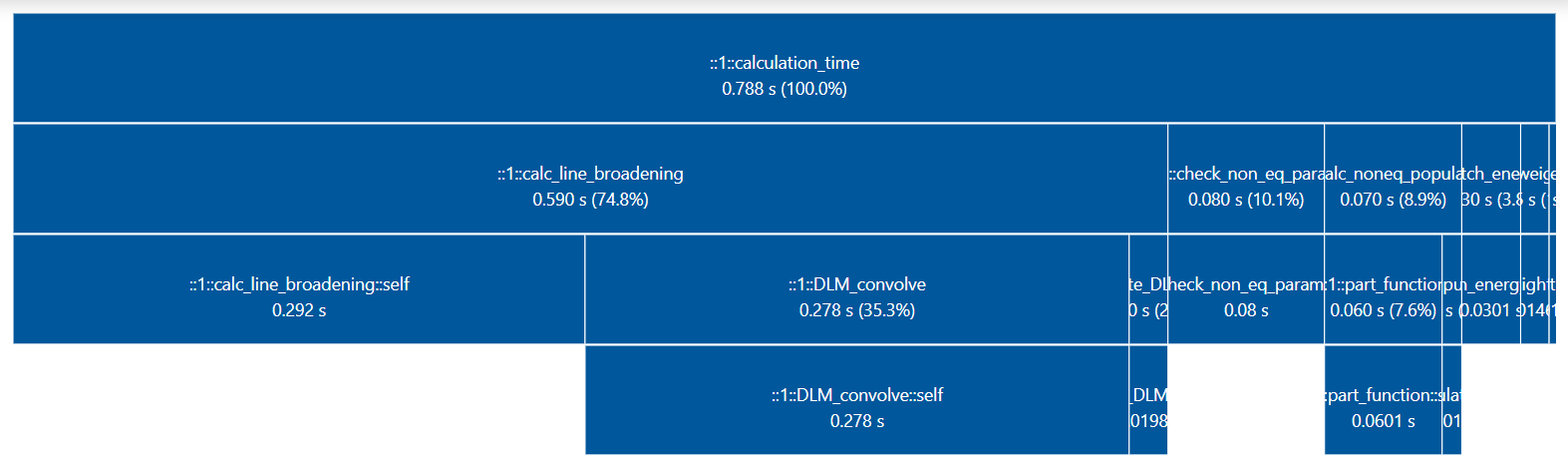### Predict Time¶

`predict_time()` function uses the input parameters like `Spectral Range`, `Number of lines`, `wstep`, `truncation` to predict the estimated calculation time for the Spectrum broadening step(bottleneck step) for the current optimization and broadening_method. The formula for predicting time is based on benchmarks performed on various parameters for different optimization, broadening_method and deriving its time complexity.

The following Benchmarks were used to derive the time complexity:

Complexity vs Calculation Time Visualizations for different optimizations and broadening_method:

See `init_database()`, which is the direct integration of `SpecDatabase` in a `SpectrumFactory`